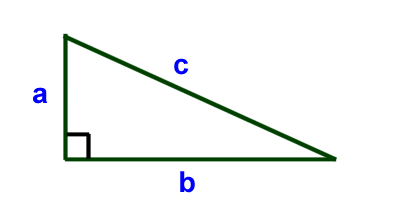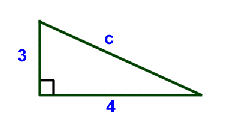# The Pythagorean Theorem

In the Sacred Numbers we have been learning about Pythagoras and sacred geometry so I thought why not write about Pythagoras’ sacred geometry theory! I hope you enjoy!

The Pythagorean Theorem helps us to figure out the length of the sides of a right triangle. If a triangle has a right angle (also called a 90 degree angle) then the following formula holds truth: a2 + b2 = c2Where a, b, and c are the lengths of the sides of the triangle and c is the side opposite the right angle. In this example, c is also called the hypotenuse (longest side of the triangle)

1) Solve for c in the triangle below: In this example a = 3 and b=4. Let’s plug those into the Pythagorean Formula. a2 + b2 = c2 32 + 42 = c2 3×3 + 4×4 = c2 9+16 = c2 25 = c x c c = 52) Solve for a in the triangle below: In this example b=12 and c= 15 a2 + b2 = c2 a2 + 122 = 152 a2 + 144 = 225 Subtract 144 from each side to get: 144 – 144 + a2 = 225 – 144 a2 = 225 – 144 a2 = 81 a = 9The Pythagorean Theorem itself is named after a Greek mathematician named Pythagoras. He came up with the theory that helped to produce this formula. The formula is very useful in solving all sorts of problems. Here is what the theorem says: In any right triangle, the area of the square whose side is the hypotenuse (remember this is the side opposite the right angle) is equal to the sum of the areas of the squares whose sides are the two legs (the two sides that meet at a right angle). This may not make a lot of sense when you first read it. Let’s show more of what the formula does and what the words say in a picture. If you take each side of a triangle, then you get the three squares shown below. The area of each square is length x width. So in this example the area of each square is a2, b2, and c2.What the theorem says is that the area of the purple square plus the area of the blue square will equal the area of the green square. That’s the same as saying: a2 + b2 = c2.

Pythagorus is a master of philosophy and silence he decided to sit in silence for five years to here the sounds of the Planets. He did and he said

“It was the greatest experience of my life.”

He is also one of the cleverest human beings on the planet to this day because of his knowledge on astronomy and numbers.

I will be writing about his life in tomorrows post, as there is a lot to cover.

### How I am Feeling

At the moment I am feeling a bit sad I don’t know why but I do. I am also feeling really happy because of the fact that I am attending this amazing mathematics retreat with some amazing people. Jain 108 (Mathemagics) especially he teaches kids that have trouble at school or kids that get home schooled sacred geometry and also things like fibonacci sequence (1,1,2,3,5,8,13,21,34, 55, goes on for ever =1.618) he is so amazing check him out!

I hope you guys have enjoyed todays blog post and if you have don’t forget to smash that like button and follow my blog is you are new around here!

Peace

## One thought on “The Pythagorean Theorem”

1.Grandad says:

Yer boring crud. Me heads hurting now. Xxx

Like